Question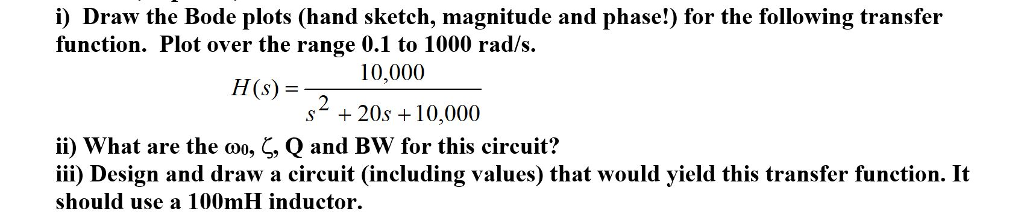We need at least 10 more requests to produce the answer.

0 / 10 have requested this problem solution

The more requests, the faster the answer.

All students who have requested the answer will be notified once they are available.

#### Earn Coins

Coins can be redeemed for fabulous gifts.

Similar Homework Help Questions
• ### Bode Plots Sketch the Bode plot magnitude and phase for each of the three open-loop transfer...

Bode Plots Sketch the Bode plot magnitude and phase for each of the three open-loop transfer functions listed below. Verify your results using the bode m function in MATLAB.(a) $$G(s)=\frac{100}{s(0.1 s+1)(0.01 s+1)}$$(b) $$G(s)=\frac{1}{(s+1)^{2}\left(s^{2}+s+9\right)}$$(c) $$G(s)=\frac{16000 s}{(s+1)(s+100)\left(s^{2}+5 s+1600\right)}$$

• ### For the following transfer function, sketch approximate straight-line Bode plots, including magnitude and phase plots. Show...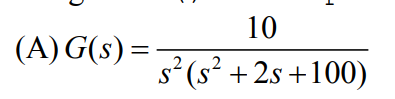For the following transfer function, sketch approximate straight-line Bode plots, including magnitude and phase plots. Show all steps clearly 10 4 (A)G(s)-7 s (s 2s +100)

• ### Sketch the approximate Bode magnitude and phase plots for the following transfer functions by hand. a....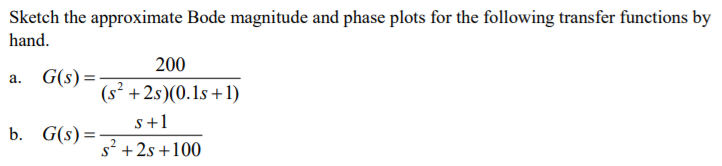Sketch the approximate Bode magnitude and phase plots for the following transfer functions by hand. a. G(s) b. G(s)- 200 (s2 +2s)(0.1s +1) s+1 s2 +2s +100

• ### For the following closed-loop transfer functions, sketch the bode plots (magnitude and phase), id...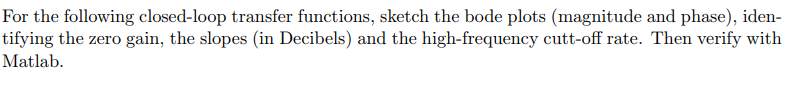For the following closed-loop transfer functions, sketch the bode plots (magnitude and phase), iden- tifying the zero gain, the slopes (in Decibels) and the high-frequency cutt-off rate. Then verify with Matlab (6) wn = 1, 〈 0.0.1, and 0.707. (8) Assuming the system of Problem 6 above, and an input of r(t) = 30sin(1000 t), use your bode plot to obtain the steady-state response For the following closed-loop transfer functions, sketch the bode plots (magnitude and phase), iden- tifying the...

• ### Sketch the Bode magnitude and phase plots for the following transfer function: G(s)=- a fimction: G(9)=...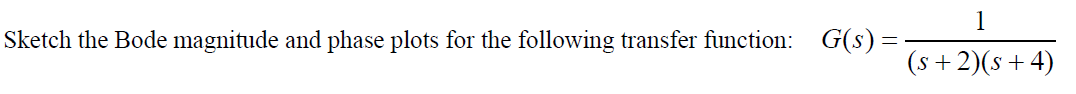Sketch the Bode magnitude and phase plots for the following transfer function: G(s)=- a fimction: G(9)= (s+2016+4) (s + 2)(+4)

• ### Please plot on semi-log scale for both magnitude and phase separately B. Sketch the Bode plots...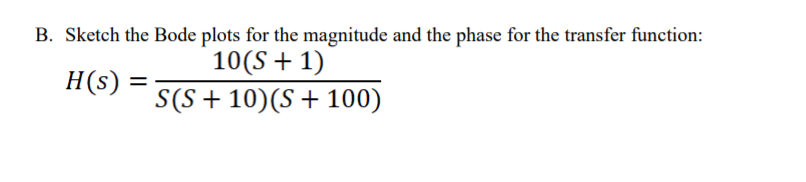Please plot on semi-log scale for both magnitude and phase separately B. Sketch the Bode plots for the magnitude and the phase for the transfer function: 10(S + 1) H(S) = S(S + 10)(8 + 100)

• ### For the following closed-loop transfer functions, sketch the bode plots (magnitude and phase), id...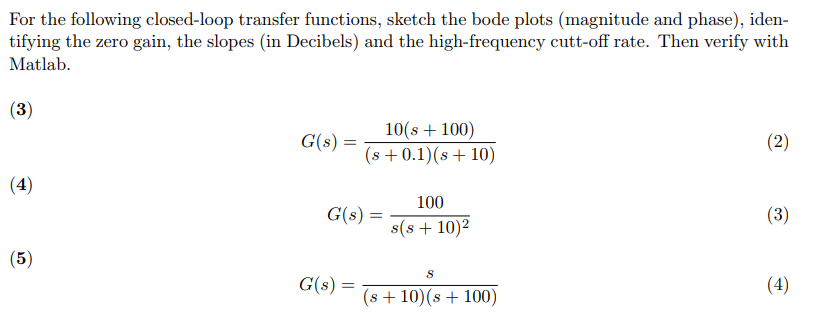For the following closed-loop transfer functions, sketch the bode plots (magnitude and phase), iden- tifying the zero gain, the slopes (in Decibels) and the high-frequency cutt-off rate. Then verify with Matlab C()101 100) s 0.1) (s 10) 100 s(s +10)2 G(s) = (56) G(s) = s+10(s+100) For the following closed-loop transfer functions, sketch the bode plots (magnitude and phase), iden- tifying the zero gain, the slopes (in Decibels) and the high-frequency cutt-off rate. Then verify with Matlab C()101 100) s...

• ### I need help with this  Bode Plots assignment (5 points) Follow the steps (a-c) described below for the following transf...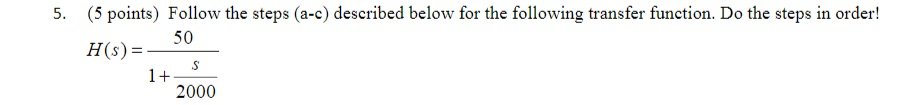I need help with this  Bode Plots assignment (5 points) Follow the steps (a-c) described below for the following transfer function. Do the steps in order! 5. H50 H(s) 2000 a. On a 'blank Bode plot' grid, plot the Bode plot straight line hand sketch approximation for magnitude for the frequency range from 1000 to ω.*1000. Be sure, though, that the corner frequency aligns with an appropriate vertical line on the grid. b. On graph paper, directly below the magnitude...

• ### 1- For each transfer function below, sketch the Bode magnitude and phase plots, a) T(s) 3040S...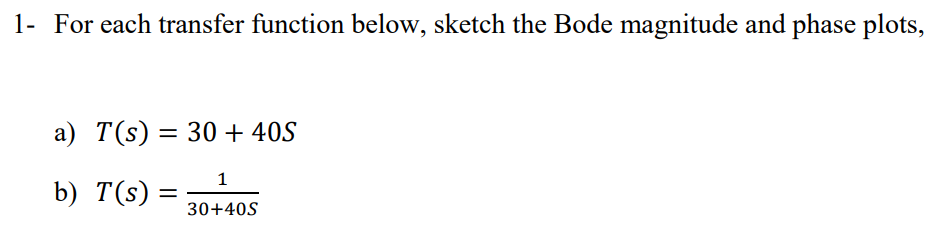1- For each transfer function below, sketch the Bode magnitude and phase plots, a) T(s) 3040S b) T(S) 30-405

• ### I need help with this  Bode Plots assignment 2. (5 points) Follow the steps (a-c) described below for the following tra...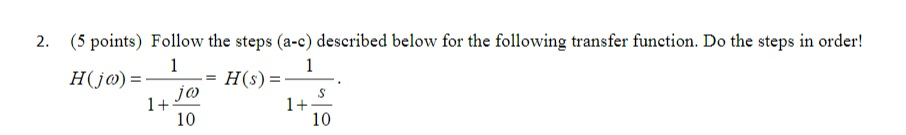I need help with this  Bode Plots assignment 2. (5 points) Follow the steps (a-c) described below for the following transfer function. Do the steps in order! 1+ 10 1+ 10 a. On a 'blank Bode plot' grid, plot the Bode plot straight line hand sketch approximation for magnitude for the frequency range from 1000 to ω.*1000. Be sure, though, that the corner frequency aligns with an appropriate vertical line on the grid. b. On graph paper, directly below the...